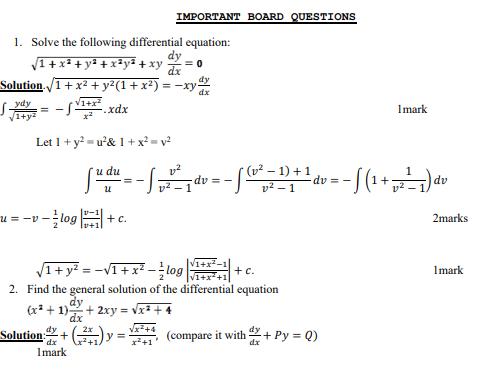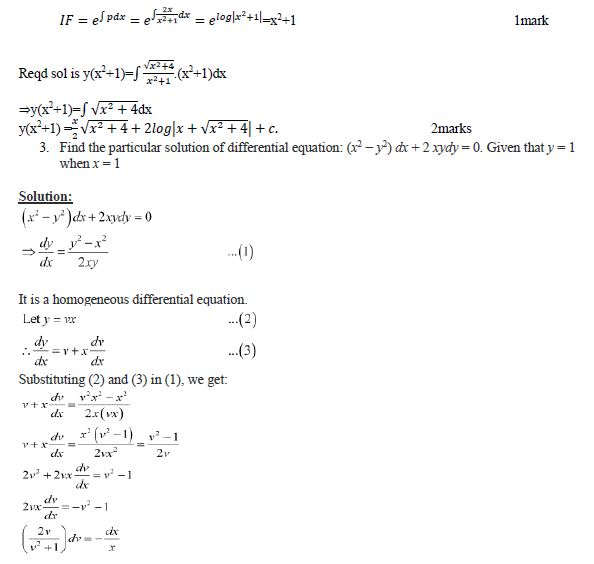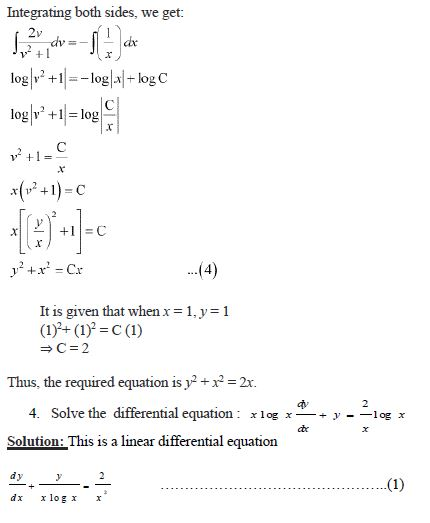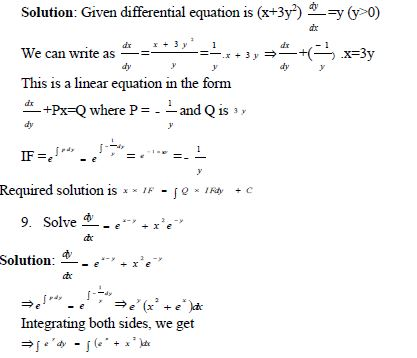# CBSE Class 12 Mathematics Differential Equation Notes

Download CBSE Class 12 Mathematics Differential Equation Notes in PDF format. All Revision notes for Class 12 Differentials Equation have been designed as per the latest syllabus and updated chapters given in your textbook for Differentials Equation in Standard 12. Our teachers have designed these concept notes for the benefit of Grade 12 students. You should use these chapter wise notes for revision on daily basis. These study notes can also be used for learning each chapter and its important and difficult topics or revision just before your exams to help you get better scores in upcoming examinations, You can also use Printable notes for Class 12 Differentials Equation for faster revision of difficult topics and get higher rank. After reading these notes also refer to MCQ questions for Class 12 Differentials Equation given our website

## Class 12 Differentials Equation Revision Notes

Class 12 Differentials Equation students should refer to the following concepts and notes for Differentials Equation in standard 12. These exam notes for Grade 12 Differentials Equation will be very useful for upcoming class tests and examinations and help you to score good marks

### Notes Class 12 Differentials Equation

Definition: An equation involving the independent variable x(say), dependent variable y(say) and the differential coefficient of dependent variable w.r.t independent variable i.e dy/dx, d2y/d2x,…. etc is called differential
equation.

Order of a differential equation is the order of the highest order derivative occurring in the differential equation.

Degree of a differential equation is the degree of highest order derivative occurring in the differential equation when the differential coefficients are made free from radicals, fractions and it is written as a polynomial in differential coefficients.

e.g(d2y/d2x)3+sin(dy/dx) = 0 here order is 2 but this differential equation can’t be written in the form of polynomial in differential coefficient Hence its degree not defined.

Linear and Nonlinear Differential Equations: A differential equation in which the dependent variable and its derivatives occur only in the first degree and are not multiplied together, is called a linear differential equation otherwise it is non-linear.

“Formation of differential Equation” To form a DE from a given equation in x and y containing arbitrary constants (parameters) –

“Initial value problem(IVP) is one in which some initial conditions are given to solve a DE”

1. Differentiate the given equation as many times as the number of arbitrary constants involved in it.
2. Eliminate the arbitrary constant from the equations of y, y’, y’’ etc.

Solution of Differential Equations-
1. Variable separable form
2. Homogenous Equations
3. Linear Differential Equations

VARIABLE SEPARABLE FORM If in the equation, it is possible to get all terms containing x and dx to one side and all the terms containing y and dy to the other, the variables are said to be separable,

Procedure to solve:
Consider the equation dy/dx
= X.Y, where X is a function of x only and Y is function of y only.
(i) Put the equation in the form 1/Y
,dy=X.dx.
(ii) Integrating both the sides we get

Homogeneous Differential Equations
A differential equation which can be expressed in the form dy/dx=f(x,y) or dx/dy=g(x,y) where, f (x, y) and g(x, y) are homogenous functions of degree zero is called a homogeneous differential equation

Steps for Solving a Homogeneous Differential Equation

1. Rewrite the differential in homogeneous form
2. Make the substitution y = vx orx = vy where v is a variable.
3. Substitute to rewrite the differential equation in terms of v and x or v and y only
4. Follow the steps for solving separable differential equations.
5. Re-substitute v = y/x or v = x / y in the final solution.

Linear Differential Equation: A first-order linear differential equation can be written in the form dy/dx+PY=Q)

where P and Q are constants or function of x only or dx/dy+Px = Qwhere P and Q are constants or function of
y only.## Tags:

Click for more Differentials Equation Study Material
 CBSE Class 12 Mathematics Differential Equation Notes

## Latest NCERT & CBSE News

Read the latest news and announcements from NCERT and CBSE below. Important updates relating to your studies which will help you to keep yourself updated with latest happenings in school level education. Keep yourself updated with all latest news and also read articles from teachers which will help you to improve your studies, increase motivation level and promote faster learning

### CBSE Term 2 Board Examinations

CBSE vide Circular No.Acad-51/2021 dated 5th July, 2021, notified that in the session 2021-2022, Board Examinations would be conducted in two terms, i.e.. Term I and Term II. This decision was taken due to the uncertainty arising out of COVID 19 Pandemic. Term I...

This is with reference to CBSE Notification No. 40/2021 dated 04.05.2021 regarding the Innovation Ambassador program – An online training program for teachers by CBSE in collaboration with Ministry of Education’s Innovation Cell (MIC) and AICTE. In view of the current...

### Heritage India Quiz 2021 2022

CBSE Heritage India Quiz is conducted every year to raise the awareness about the preserving human heritage, diversity and vulnerability of the India's built monuments and heritage sites. It is an attempt of the Board to motivate the future generations of this country...

### Celebration of Matribhasha Diwas Mother Language day

UNESCO has declared 21st February of every year to be celebrated as International Mother Language day to promote dissemination of Mother Language of all, create awareness of linguistic and cultural traditions and diversity across the world and to inspire solidarity...

### CBSE Science Challenge 2021 22

Science is inexplicably linked with our lives and helps us to understand the world around us better. Scientific and technological developments contribute to progress and help improve our standards of living. By engaging with this subject, students learn to think, solve...

### Online courses for classes XI and XII offered by NCERT

Ministry of Education (MoE), Government of India has launched a platform for offering Massive Open Online Courses (MOOCs) that is popularly known as SWAYAM (Study Webs of Active learning for Young Aspiring Minds) on 9 th July, 2017. NCERT now offers online courses for...